# 什么是树?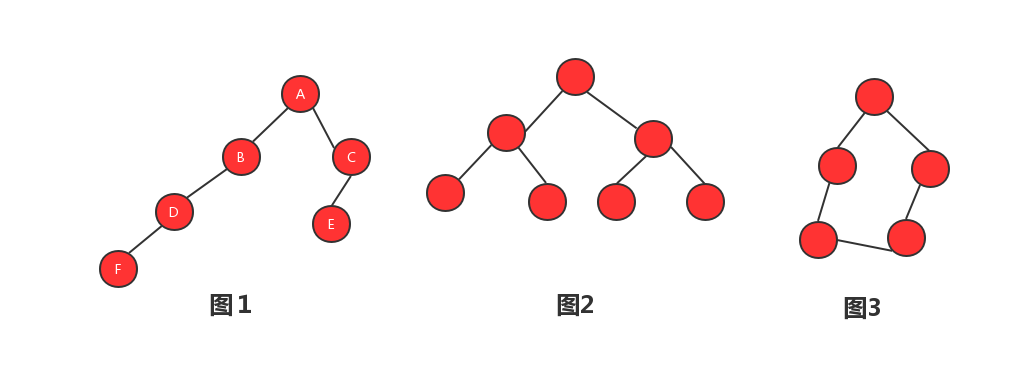：节点距离根节点的距离，从１开始计数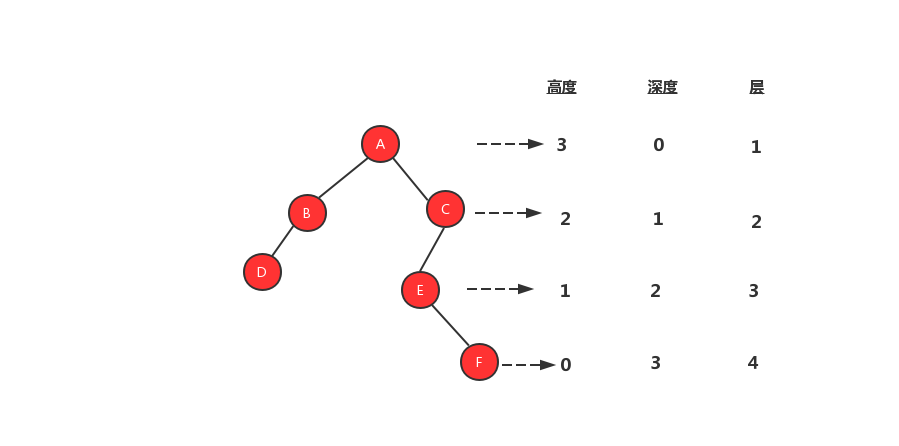# 二叉树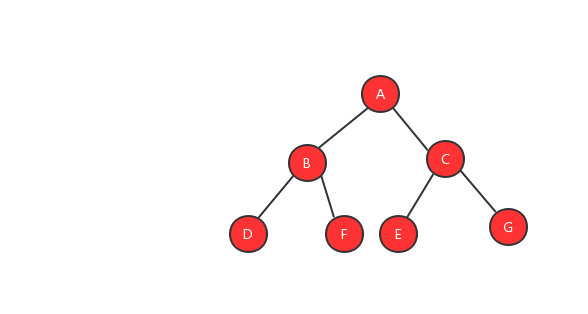#### １、完全二叉树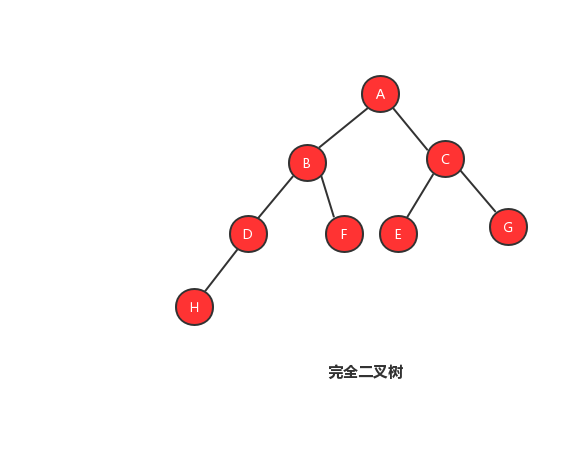#### ２、非完全二叉树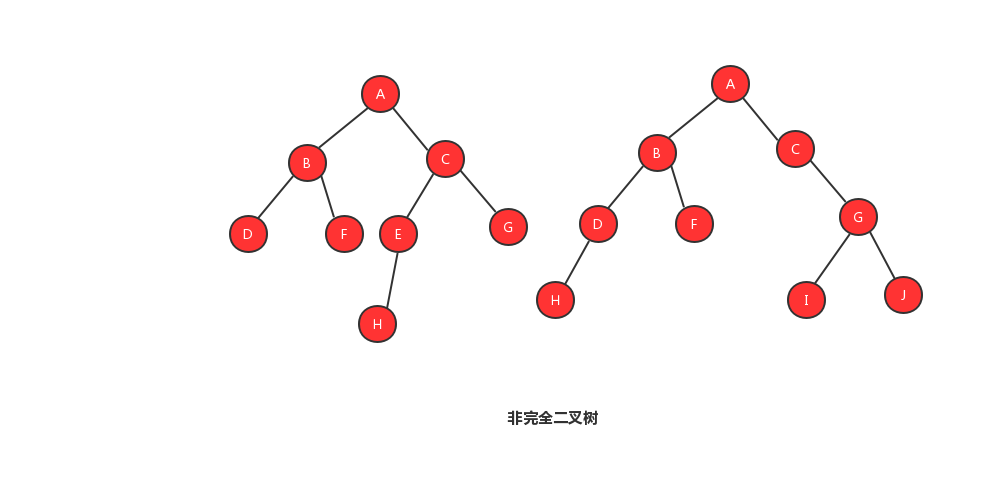## 二叉树的存储模式

### 二叉链式存储法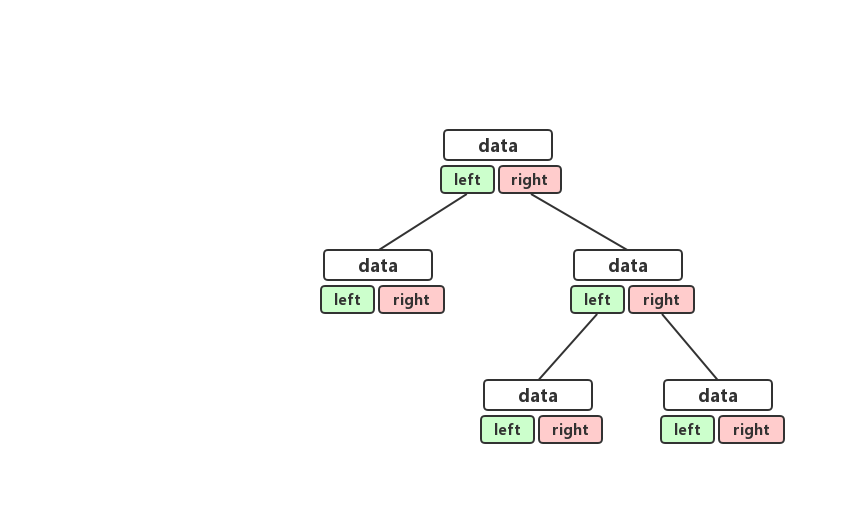### 顺序存储法

###### 完全二叉树顺序存储法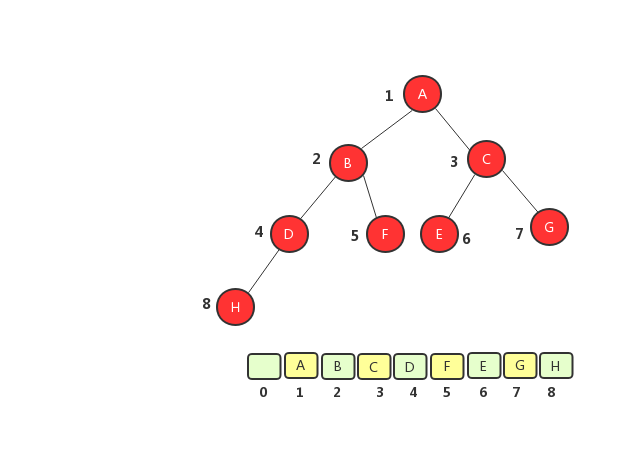###### 非完全二叉树顺序存储法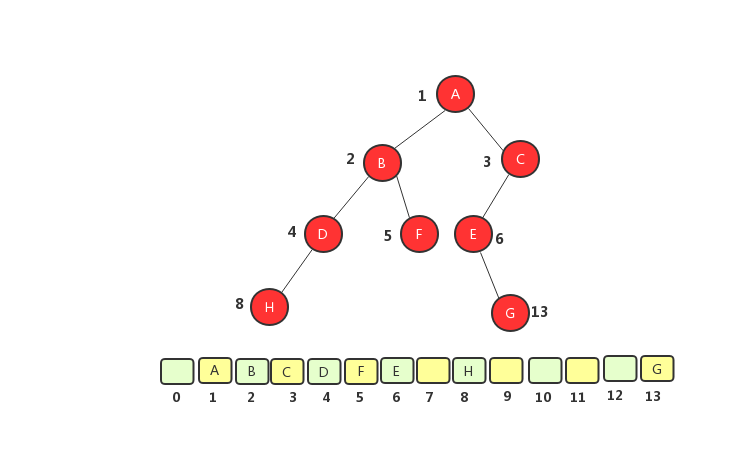## 二叉树遍历

/**
* 定义一棵树
*/
public class TreeNode {
// 存储值
public int data;
// 存储左节点
public TreeNode left;
// 存储右节点
public TreeNode right;

public TreeNode(int data) {
this.data = data;
}
}


public static TreeNode buildTree() {
// 创建测试用的二叉树
TreeNode t1 = new TreeNode(1);
TreeNode t2 = new TreeNode(2);
TreeNode t3 = new TreeNode(3);
TreeNode t4 = new TreeNode(4);
TreeNode t5 = new TreeNode(5);
TreeNode t6 = new TreeNode(6);
TreeNode t7 = new TreeNode(7);
TreeNode t8 = new TreeNode(8);

t1.left = t2;
t1.right = t3;
t2.left = t4;
t4.right = t7;
t3.left = t5;
t3.right = t6;
t6.left = t8;

return t1;
}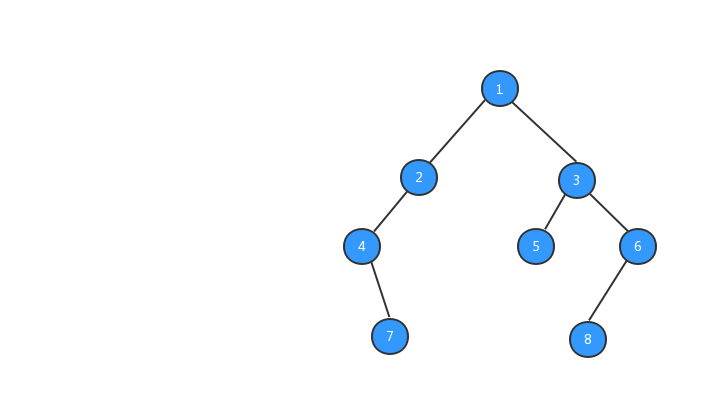### 前序遍历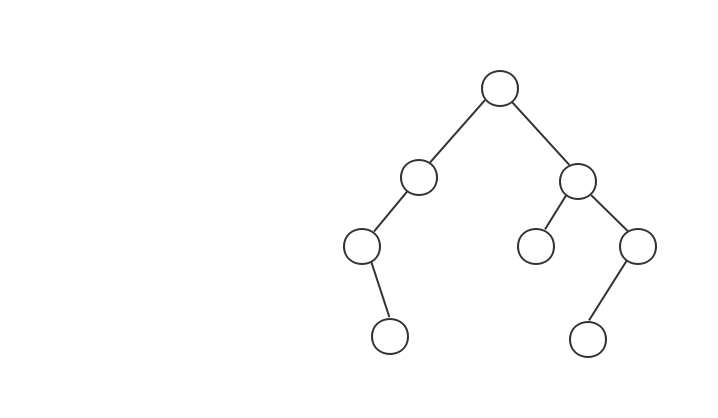// 先序遍历，递归实现 先打印本身，再打印左节点，在打印右节点
public static void preOrder(TreeNode root) {

if (root == null) {
return;
}
// 输出本身
System.out.print(root.data + " ");
// 遍历左节点
preOrder(root.left);
// 遍历右节点
preOrder(root.right);
}

### 中序遍历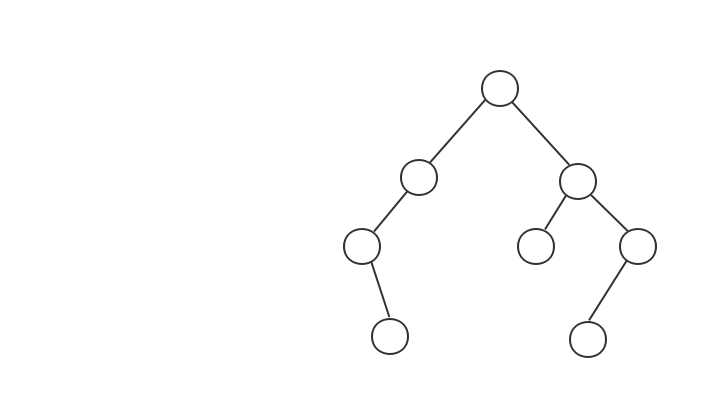// 中序遍历 先打印左节点，再输出本身，最后输出右节点
public static void inOrder(TreeNode root) {
if (root == null) {
return;
}
inOrder(root.left);
System.out.print(root.data + " ");
inOrder(root.right);
}

### 后序遍历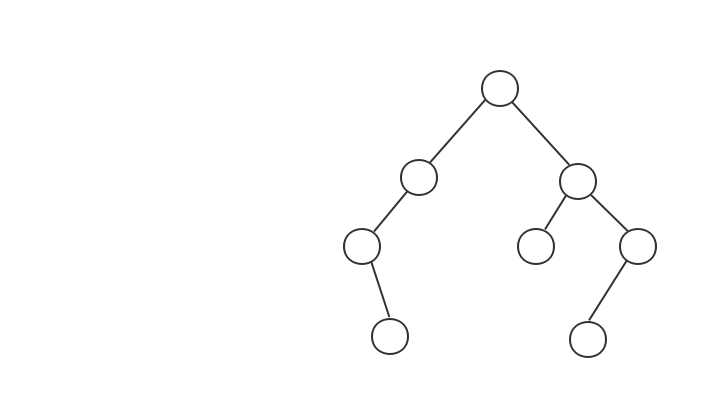// 后序遍历 先打印左节点，再输出右节点，最后才输出本身
public static void postOrder(TreeNode root) {
if (root == null) {
return;
}
postOrder(root.left);
postOrder(root.right);
System.out.print(root.data + " ");
}

# 二叉查找树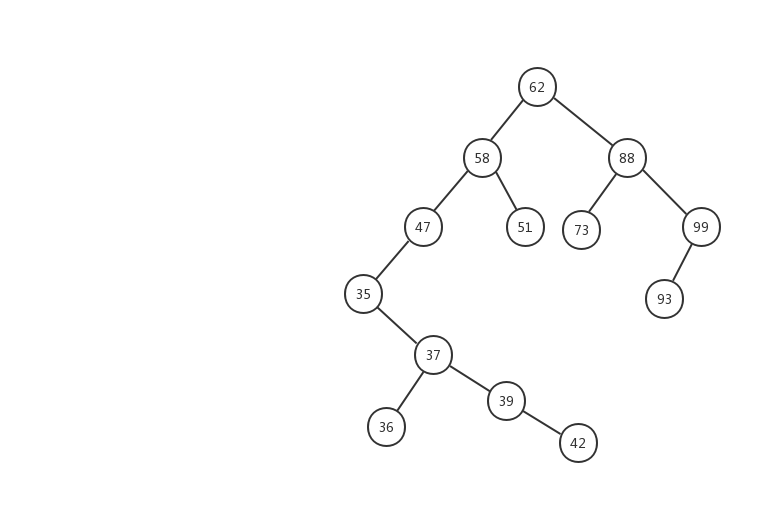## 二叉查找树的查找操作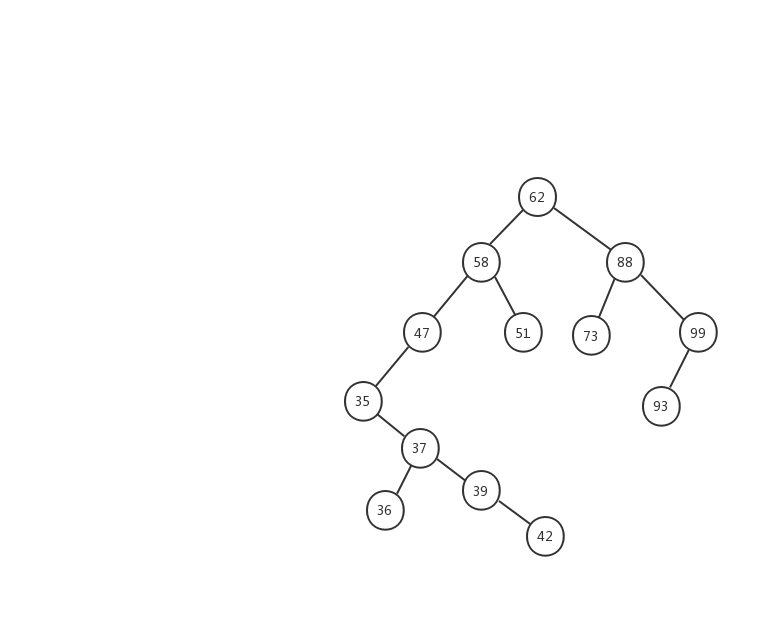• 1、先用 37 跟 62 比较，37 < 62 ，在左子树中继续查找
• ２、左子树的节点值为 58，37 < 58 ，继续在左子树中查找
• ３、左子树的节点值为 47，37 < 47，继续在左子树中查找
• ４、左子树的节点值为 35，37 > 35，在右子树中查找
• ５、右子树中的节点值为 37，37 = 37 ，返回该节点

/**
* 根据值查找树
* @param data　值
* @return
*/
public TreeNode find(int data) {
TreeNode p = tree;
while (p != null) {
if (data < p.data) p = p.left;
else if (data > p.data) p = p.right;
else return p;
}
return null;
}

## 二叉查找树的插入操作• 1、63 > 62 ，在树的右子树继续查找.
• 2、63 < 88 ，在树的左子树继续查找
• 3、63 < 73 ,因为 73 是叶子节点，所以 63 就成为了 73 的左子树。

/**
* 插入树
* @param data
*/
public void insert(int data) {
if (tree == null) {
tree = new TreeNode(data);
return;
}

TreeNode p = tree;

while (p != null) {
// 如果值大于节点的值，则新树为节点的右子树
if (data > p.data) {
if (p.right == null) {
p.right = new TreeNode(data);
return;
}
p = p.right;
} else { // data < p.data
if (p.left == null) {
p.left = new TreeNode(data);
return;
}
p = p.left;
}
}
}

## 二叉查找树的删除操作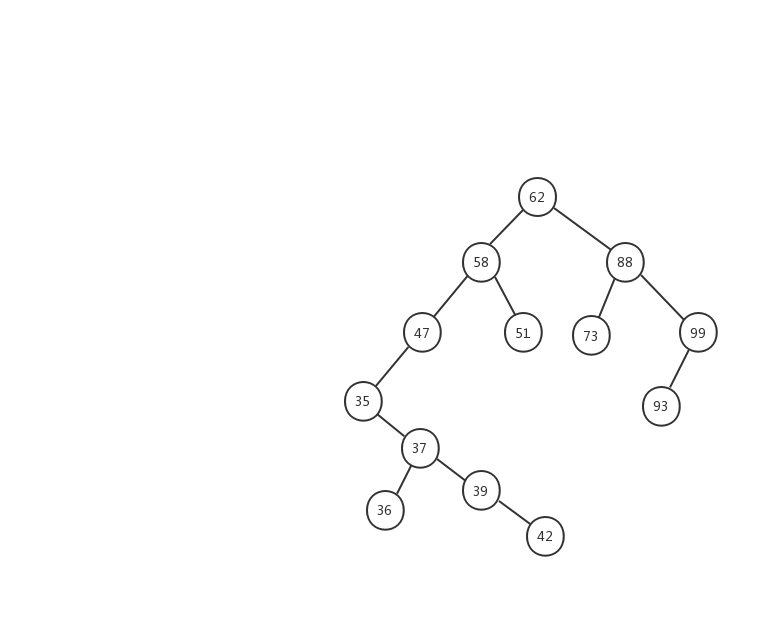public void delete(int data) {
TreeNode p = tree; // p指向要删除的节点，初始化指向根节点
TreeNode pp = null; // pp记录的是p的父节点
while (p != null && p.data != data) {
pp = p;
if (data > p.data) p = p.right;
else p = p.left;
}
if (p == null) return; // 没有找到

// 要删除的节点有两个子节点
if (p.left != null && p.right != null) { // 查找右子树中最小节点
TreeNode minP = p.right;
TreeNode minPP = p; // minPP表示minP的父节点
while (minP.left != null) {
minPP = minP;
minP = minP.left;
}
p.data = minP.data; // 将minP的数据替换到p中
p = minP; // 下面就变成了删除minP了
pp = minPP;
}

// 删除节点是叶子节点或者仅有一个子节点
TreeNode child; // p的子节点
if (p.left != null) child = p.left;
else if (p.right != null) child = p.right;
else child = null;

if (pp == null) tree = child; // 删除的是根节点
else if (pp.left == p) pp.left = child;
else pp.right = child;
}

# 最后08-032702
11-0257908-221万+
11-15673
03-042万+
11-2379
03-106920
09-061万+
03-301万+
12-286920
11-132204
04-21875
08-29159
02-082万+
04-051万+
12-31
©️2020 CSDN 皮肤主题: 编程工作室 设计师:CSDN官方博客